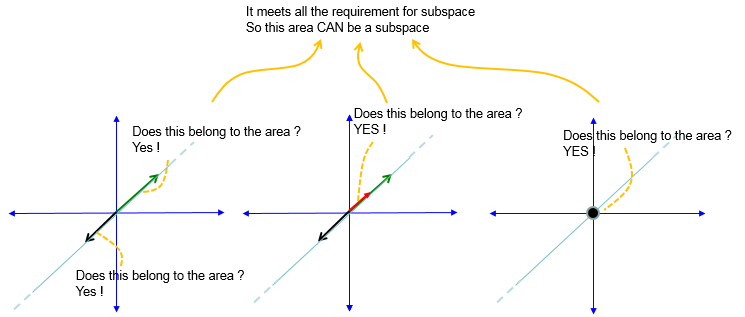Engineering Math - Quick Reference                                 Home : www.sharetechnote.com Vector Space, SubSpace, Null Space   Vector Space means a space that can be covered by adding any two or more vectors or by multiplying with scaler. These two original vectors (vectors being used for the operation) should also belong to the same space and the result of the operation should also be within the space. It is similar concept to a set like Integer Set or Real Set etc in number system. For example, let's suppose you have a 2-D plane as indicated in blue axis shown below. As you see, we assume that the axis goes to infinity even though we cannot draw such a plane with infinite size. And let's assume that you draw two vectors a and b. These two factor belong to the 2-D plane (let's call this as 'blue plane' now). Now let's take the sum of vector a and b and you get another vector c. As you see, the vector c also within the blue plane. Now multiply a constant to the two vector (as in green vector). Suppose you are doing these operations for any length of a and b with infinite different ways, then the resulting vectors will cover all the points on blue plane. So this blue plane can be a vector space. Since we take the real vectors in 2D real number plane, this vector space is called R^2 space. If you take this operation in 3D axis, it will be called R^3 space.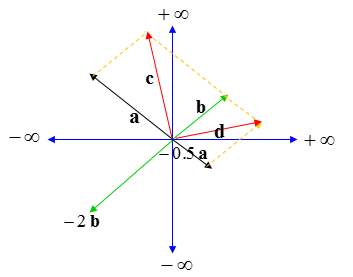Sub Space means a space that can be covered by adding any two or more vectors or by multiplying with scaler. These two original vectors (vectors being used for the operation) should also belong to the same sub space and the result of the operation should also be within the space. If I represent this in mathematical symbols, it would be as follows. To be a Subspace, it has to meet all of these criteria. If only one of the criterial is not met, it cannot be a Sub space.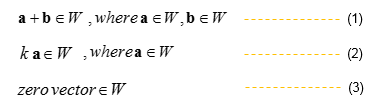Let's try with some examples for more intuitive understanding. This idea came from What is a Subspace? on YouTube.   Here goes the first example. Let's suppose you take a small area as shown below. Can this rectangular area be a sub space ?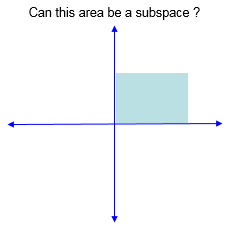This cannot be a subspace since you can easily find two vectors, sum of which gets out of the area. So this is violation of condition 1.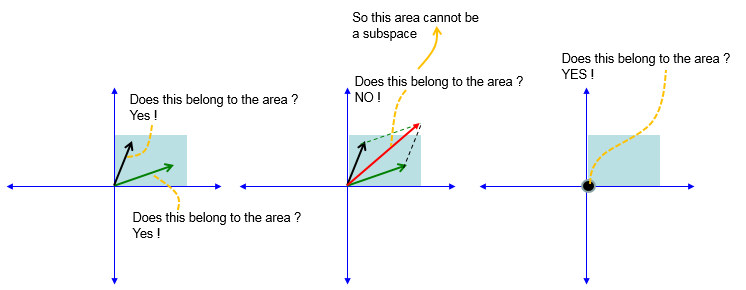Here goes another example. Let's suppose you take a straight line extending to infinite range. Note that this line is off the origin (0,0). Can this line be a sub space ?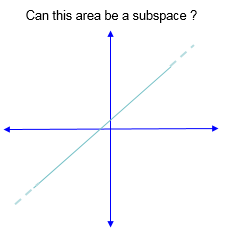Check with each condition as below. You notice that zero vector does not belong to this line. So this line cannot be a sub space.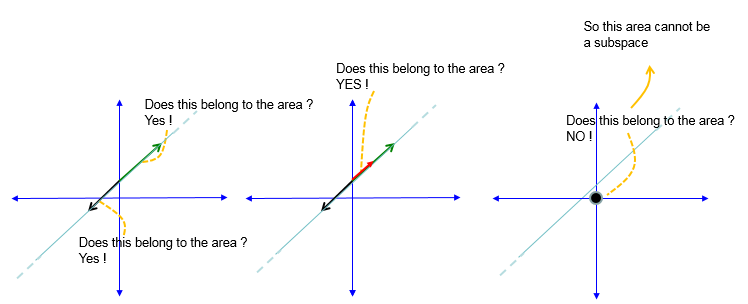Now let's see another example as shown below. In this example,  we have a stright line passing through the origin of the axis.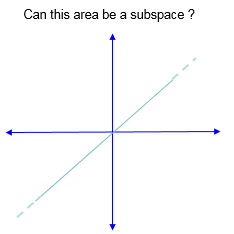Check with each condition as below. Now you see all the check points passes the criteria (one check points that is not shown here is about scaling, but you can easily confirm that if you scale green or black vector, the resulting vector still be sitting on the line) So this line cannot be a sub space.